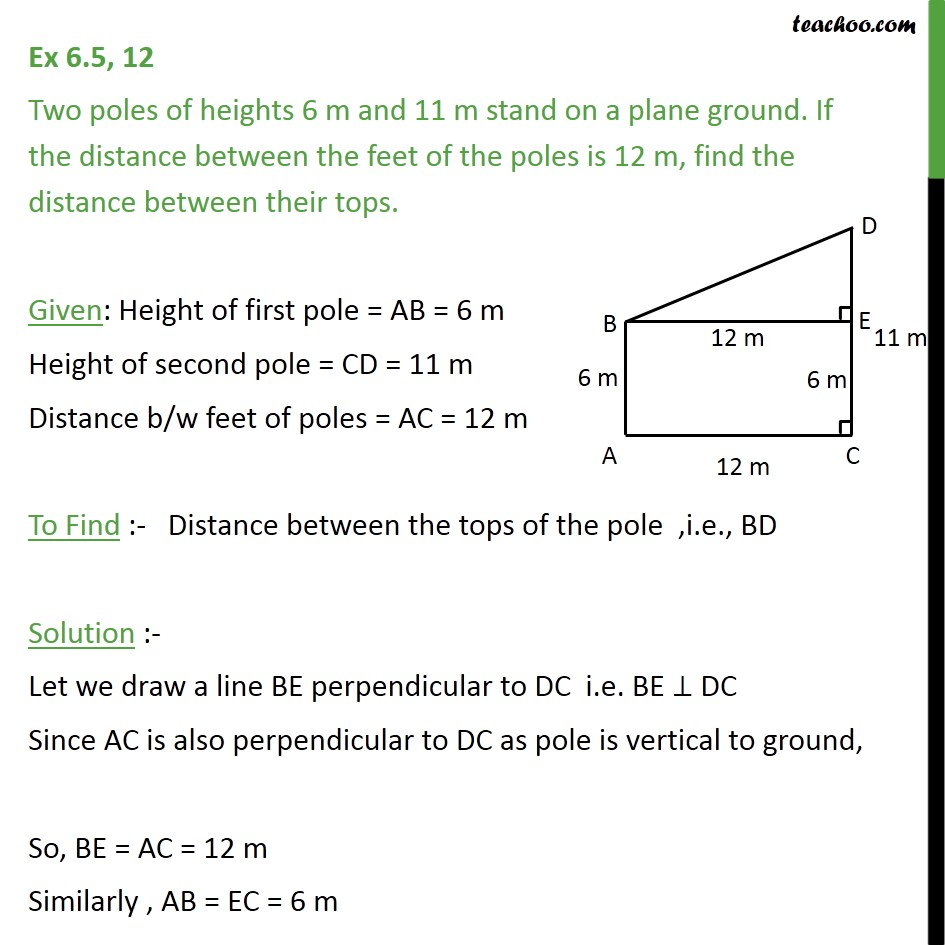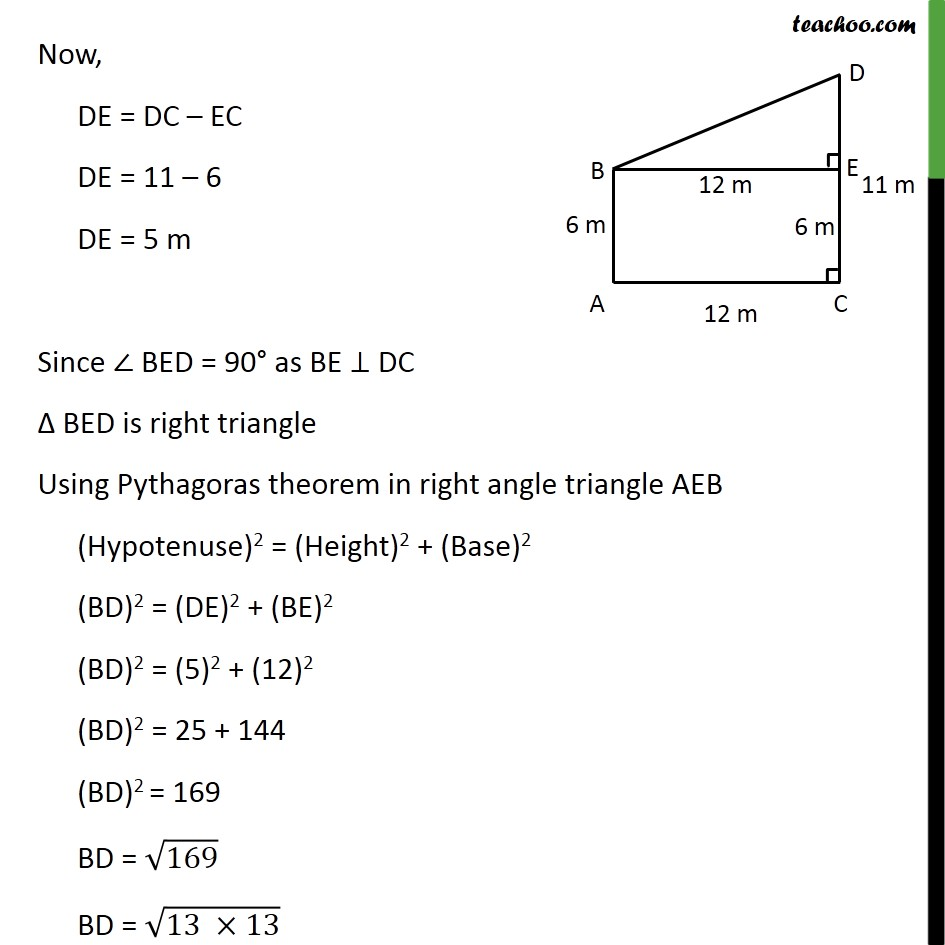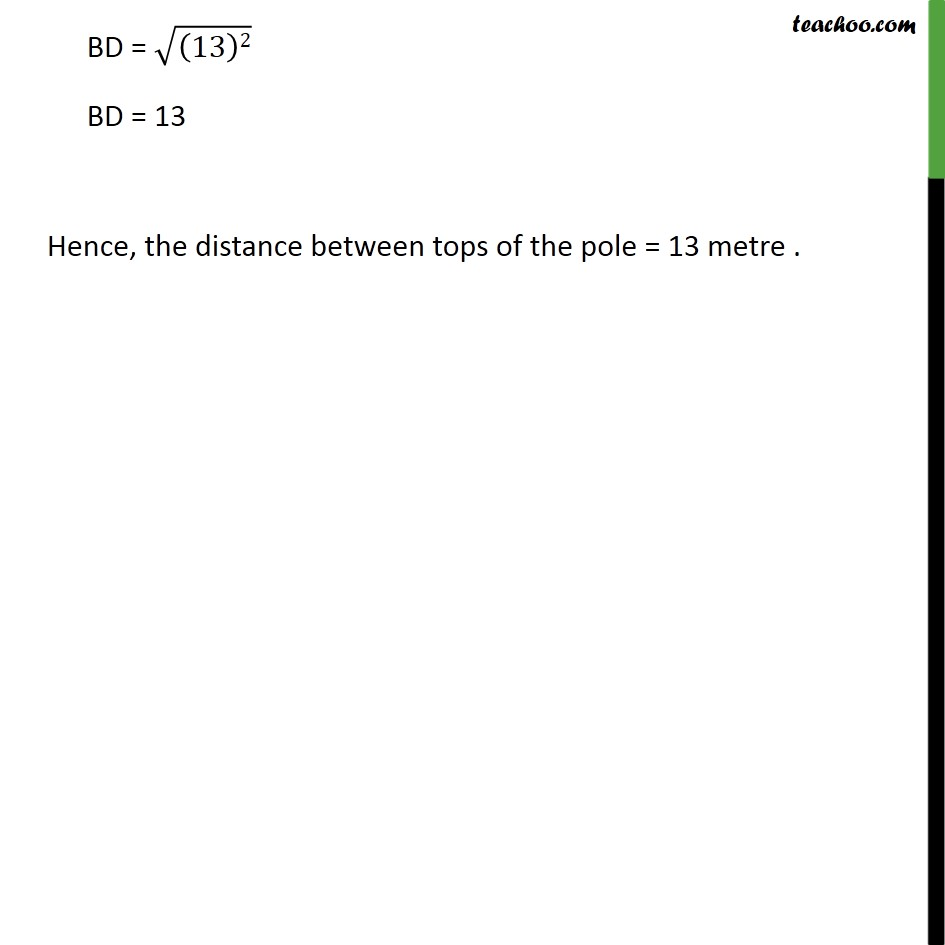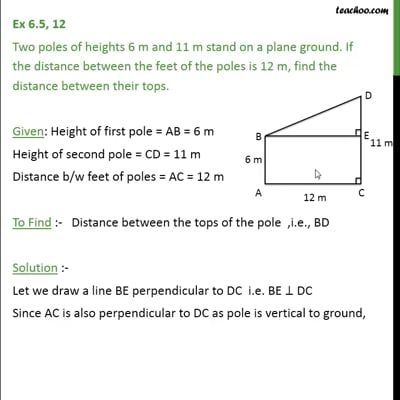Chapter 6 Class 10 Triangles

Class 10
Important Questions for Exam - Class 10This video is only available for Teachoo black users

Introducing your new favourite teacher - Teachoo Black, at only ₹83 per month

### Transcript

Ex 6.5, 12 Two poles of heights 6 m and 11 m stand on a plane ground. If the distance between the feet of the poles is 12 m, find the distance between their tops. Given: Height of first pole = AB = 6 m Height of second pole = CD = 11 m Distance b/w feet of poles = AC = 12 m To Find :- Distance between the tops of the pole ,i.e., BD Solution :- Let we draw a line BE perpendicular to DC i.e. BE DC Since AC is also perpendicular to DC as pole is vertical to ground, So, BE = AC = 12 m Similarly , AB = EC = 6 m Now, DE = DC EC DE = 11 6 DE = 5 m Since BED = 90 as BE DC BED is right triangle Using Pythagoras theorem in right angle triangle AEB (Hypotenuse)2 = (Height)2 + (Base)2 (BD)2 = (DE)2 + (BE)2 (BD)2 = (5)2 + (12)2 (BD)2 = 25 + 144 (BD)2 = 169 BD = 169 BD = (13 13) BD = ((13)2) BD = 13 Hence, the distance between tops of the pole = 13 metre .